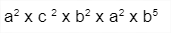# Exponents And Powers Class 8 Worksheet

On this page, you will find methods to understand exponents and powers of values, and also a few practice questions designed to help you master this ability.

## What is the “‘Exponents And Powers Class 8 Worksheet”?

The Exponents And Powers Class 8 Worksheet is made to assist learners to trigger their knowledge and understanding of concepts through math activities, word problems, assessments, and math worksheets. It will help them to create their practice questions and solve them.

## How will the “Exponents And Powers Class 8 Worksheet” help you?

This worksheet will help you understand exponents and powers with reasoning skills accompanied by logic.

### Instructions on how to use the “Exponents And Powers Class 8 Worksheet”

Use this worksheet to understand the step-by-step method for finding exponents and powers and practice your learned concepts by using the practice question given below in the worksheet.

A word problem is also given after the practice questions.

At the end of the worksheet, a reflective section is designed to make students think and create their own questions and word problems.

Lastly, it will provoke students to think and develop a unique way of finding exponents and powers

### Conclusion

The step-by-step method will help you in dealing with exponents and powers-related questions. It will give you an understanding of how to write solutions involving these two terms and form a link between exponents and mathematical operators.

## Exponents And Powers

Let’s review some concepts before starting the procedure.

Exponent:  Powers or indices

Power: A power is represented with a base number and an exponent

## Exponents And Powers

Examples
Write the question first part

1. Express each of the following numbers using the exponential notation:

Solution:

(iii)Solution:-

The given question can be expressed in the exponential form as

## Exponents and powers class 8 worksheet

Name the Terms:
Write the names of given values in blocks.

Applying:
Solve The following

Word Problem:

If a newborn bear weighs 4 kg, calculate how many kilograms a five-year-old bear weighs if its weight increases by the power of 2 in 5 years.